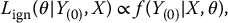# 15Missing Not at Random Models

## 15.1 Introduction

The examples and methods in Chapters 7–14 were based on the ignorable likelihood:

(15.1)regarded as a function of the parameter θ for fixed observed data Y(0); in (15.1), X represents fully observed covariates, and f(Y(0)|X, θ) is obtained by integrating the missing data Y(1) out of the density f(Y|X, θ) = f(Y(0), Y(1)|X, θ). In Chapter 6, we showed that sufficient conditions for basing inference about θ on (15.1), rather than the full likelihood from a model for Y and M given X, are that (i) the missing data are missing at random (MAR) and (ii) the parameters θ and ψ are distinct, as defined in Section 6.2. In this chapter, we consider situations where the missingness mechanism is missing not at random (MNAR), and valid ML, Bayesian, and multiple-imputation (MI) inferences generally need to be based on the full likelihood:

(15.2)regarded as a function of the parameters θ, ψ for fixed observed data Y(0) and missingness pattern M; here f(Y(0), M|X, θ, ψ) is obtained by integrating Y(1) out of the joint density f(Y, M|X, θ, ψ) based on a joint model for Y and M given X.

Two main approaches for formulating MNAR models can be distinguished. We consider them for situations where the units' values of M and Y are modeled as independent ...

Get Statistical Analysis with Missing Data., 3rd Edition now with the O’Reilly learning platform.

O’Reilly members experience live online training, plus books, videos, and digital content from nearly 200 publishers.